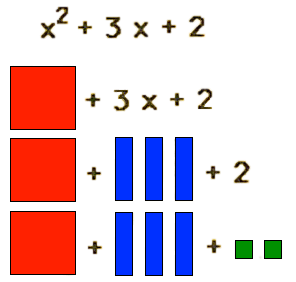# Math DictionaryTo add is to push together two or more values combining them to create a new value.

algebra -Algebra is the math strand that uses symbols to represent known and unknown numbers. The known numbers have a fixed value and the unknown numbers, represented by letters, have no fixed values. The objective of algebra is to find the value of unknown numbers. In algebra, you equate same with same to find missing values.

base ten blocks -Base-ten blocks are physical blocks used to represent numbers. We can build numbers with base-ten blocks. Base-ten blocks have different blocks to represent the different places such as the hundreds, tens, and one's place. Some base-ten blocks have multi-one, multi-ten, and multi-hundred blocks.

I Don’t Know -

I don't know is a number that we cannot count. We call I don't know numbers variables and name them by letters of the alphabet like x, y, and z.

Here are some examples of I don't know numbers:
• I don't know x, y, z
• I don't know square x², y², z²
• I don't know cube x³, y³, z³
Number Bases -

A number base is the integer building block used to build numbers. For example, we use base-ten integer blocks to build numbers in base-ten. The building blocks of all real numbers are integer blocks. The base tells us the kind of integer blocks we need to use and denotes the name of the number system.

You will likely be familiar with base-ten integer blocks. The base-ten number system is the most common number-building system. But we can use other integer blocks to build numbers too.

• The base-ten decimal counting system uses 0, 1, 2, 3, 4, 5, 6, 7, 8, 9 - base₁₀
• The base-nine nonary counting system uses 0, 1, 2, 3, 4, 5, 6, 7, 8 - base₉
• The base-eight octal counting system uses 0, 1, 2, 3, 4, 5, 6, 7 -base₈
• The base-seven septenary counting system uses 0, 1, 2, 3, 4, 5, 6 - base₇
• The base-six senary counting system uses 0, 1, 2, 3, 4, 5 - base₆
• The base-five quinary counting system uses 0, 1, 2, 3, 4 - base₅
• The base-four quarternary counting system uses digits 0, 1, 2, 3 - base₄
• The base-three ternary counting system uses 0, 1, 2 - base₃
• The base-two binary counting system uses 0, 1 - base₂
• The base-one unary counting system - we will come back to this - base₁.
• The base-zero counting system - we will come back to this - base₀.

The place of each base tells us what power of the base we are in, and the value tells us how many we have in that place. For example, in base ten, we have powers of ten in the places. Increasing by powers of ten as we move to the left and decreasing as we move to the right.

Radix is the number of digits used in a positional number system before moving to the next digit's place.

• In the base-ten number system, ten digits are used in each place - 0, 1, 2, 3, 4, 5, 6, 7, 8, 9; therefore the base-ten number system's radix is ten.
• Base nine uses nine digits in each place - 0, 1, 2, 3, 4, 5, 6, 7, 8 - radix of 9.
• Base eight uses eight digits in each place - 0, 1, 2, 3, 4, 5, 6, 7 - radix of 8.
• Base seven uses seven digits in each place - 0, 1, 2, 3, 4, 5, 6 - radix of 7.
• Base six uses six digits in each place - 0, 1, 2, 3, 4, 5 - radix of 6.
• Base five uses five digits in each place - 0, 1, 2, 3, 4 - radix of 5.
• Base four uses four digits in each place - 0, 1, 2, 3 - radix of 4.
• Base three uses three digits in each place - 0, 1, 2 - radix of 3.
• Base two uses two digits in each place - 0, 1 - radix of 2.
• Base one - we will come back to.
• Base zero - we will come back to.

This math dictionary is a beginning work in progress. Please come back often to see new words and terms added.